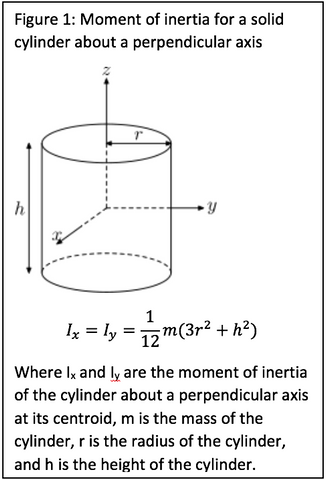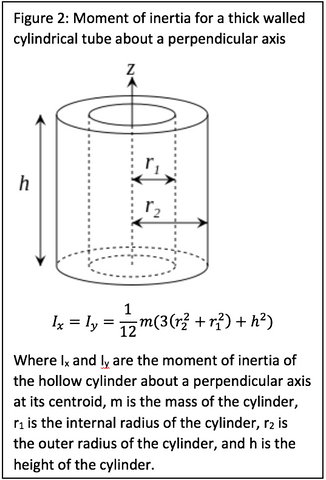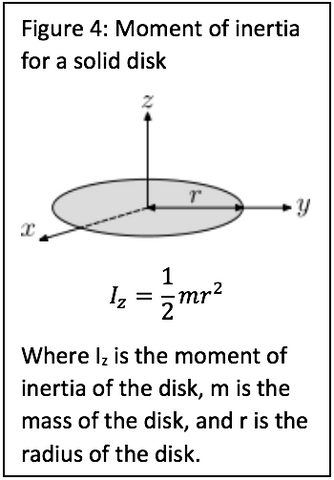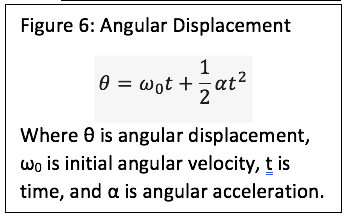Search

# Dynamic Resistance Analysis

The Waveweight is a new workout device that utilizes the dynamic nature of water to provide a more complete workout in less time than traditional weights. While the ever changing weight distribution that the Waveweight provides is the key to its effectiveness, some of its beneficial attributes can be analyzed using traditional, solid-body equations. In order to complete meaningful analysis without involving high level fluid dynamics or needlessly overcomplicating the model, the following assumptions have been made:

1. All water in the Waveweight tubing acts as a solid mass.
2. There is no water within the Waveweight crossbars.
3. The Waveweight water tube caps are massless - all mass exists within tube walls and crossbars.

To examine the use of a Waveweight, both empty and with various amounts of water inside, during a workout, we first need to understand the concepts of torque and moment of inertia.

Torque is defined as a twisting force that tends to cause rotation. For example, torque in everyday life is the act of turning a car steering wheel or twisting a screw driver. In this analysis, we will be looking at the torque required to turn the Waveweight in a similar manner as one would turn a plate weight when doing the “bus driver” exercise.

The “bus driver” exercise consists of holding a weight out at arm’s length then slowly rotating the weight by ninety degrees then rotating back to the starting position. This motion is repeated both clockwise and counter-clockwise until the desired number of reps is achieved.

Moment of inertia is defined as a quantity expressing a body's tendency to resist angular acceleration. In simpler terms, it is a measurement of how difficult it is to start or stop something from spinning. One example of moment of inertia can be experienced when riding a bike. Large, heavy tires, such as those found on mountain bikes, take much more energy to start spinning than slimmer, lighter tires that are found on road bikes. In the case of the Waveweight, its unique shape and distribution of mass causes it to act like a much heavier weight when used in rotational exercises such as the “bus driver” mentioned earlier.

An empty Waveweight weighs eleven pounds. Using the equations for moment of inertia for a solid cylinder (Figure 1), moment of inertia for a hollow cylinder (Figure 2), and the Parallel Axis Theorem (Figure 3), the total moment of inertia for an empty Waveweight is found to be 22.78 lb·ft2. For comparison, the moment of inertia of an average 45 pound, 17.5 inch diameter disk weight, found by using the moment of inertia equation for a solid disk (Figure 4), is 11.96 lb·ft2.What this means is that the Waveweight is almost twice as difficult to rotate in the “bus driver” when held by the innermost grips as a 45 pound disk weight even though it weighs one quarter as much.

If we rearrange the moment of inertia for a solid disk equation to solve for mass, we get the following equation:By plugging in the moment of inertia of an empty Waveweight, and use the same 17.5 inch disk diameter, we find that the equivalent solid disk weight required comes out to 85.70 pounds.

Adding water to the Waveweight further increases the difficulty of rotation. Just one pound of water to each side of the Waveweight increases the equivalent disk weight needed to provide the same resistance to by an average of 4.19 pounds.Increasing the moment of inertia also causes the torque required to rotate the object to increase as well (Figure 5).Using the “bus driver” exercise as an example again, the average time for a single ninety degree rotation of a 22 pound weight is around 0.425 seconds. The rotational acceleration that is needed for this motion can be found by rearranging angular displacement formula (Figure 6):Since the exercise begins and ends with the weight not rotating, there is no initial angular velocity so the equation reduces to:The last thing to consider is that the weight must be spun up for the first half (45°) of the rotation and then slowed down to stop in the second half (45°) in order to be stationary at a quarter turn (90°). This means the time used must be divided by two.

Plugging all of these numbers in produces an acceleration of 34.78 rad/s2 which translates to 6.34 ft·lbs of torque to perform the “bus driver” exercise using a standard 22 pound disk weight.

Using the Waveweight without any water for the same exercise requires 26.7 ft·lbs of torque – over four times as much torque as in the above example.

Again, by adding water to the Waveweight, the difficulty of rotation is greatly increased. In order for the assumptions that make this analysis possible to remain true, the starting position of the Waveweight must be altered when compared to a standard “bus driver” exercise, however, the concept remains the same. Instead of starting with both hands side by side, the Waveweight must begin in a vertical position so the water remains at the bottom of the tubing. The position can be seen in Figure 7.From this modified position with just two pounds of water in each side of the Waveweight, the torque required to duplicate the original “bus driver” motion follows the curve shown at the top of the following page.At the very start of the rotation, the torque required is the same as if the Waveweight were empty, 26.7 ft·lbs. However, during the first half of rotation, the torque required to maintain constant rotational acceleration must increase in order to counteract the opposing torque of gravity pulling the water back down up to a maximum of 31.8 ft·lbs. Conversely, in order to come to a complete stop at horizontal, the torque required decreases dramatically to 21.6 ft·lbs then slowly tapers out to 19.6 ft·lbs. This is also due to gravity since it is helping to slow the rotation of the Waveweight during the second half of the rotation.

This example helps demonstrate the dynamic exercise that the Waveweight provides. The continually changing torque requirements stress the body in more varied ways than can be accomplished using standard weights.As stated at the beginning of this analysis, fluid dynamics were not considered in order to reduce the complexity of the math required. However, it has been demonstrated that the Waveweight inherently provides a dynamic exercise experience. The addition of fluid dynamic analysis would only further prove the ever changing physical output that the Waveweight is designed to produce. By harnessing the dynamic nature of water to give its user a workout that is more vigorous, real-to-life, and applicable to everyday scenarios than traditional solid weights, the Waveweight is as versatile and creative as its user.